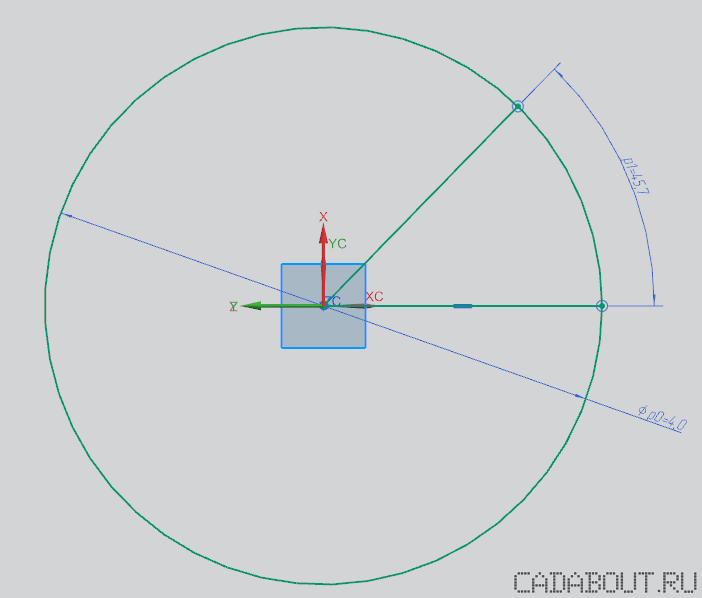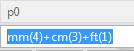# Siemens NX Jet Hint: Converting Angular, Linear and other Units using Expressions

By | 09/29/2016

You probably had facing a problem of  units conversion or dual dimensioning while working in Siemens NX. If so – then this hint would be helpful for you.

Siemens NX has an option to convert units within expressions using special functions. Let’s create a simple Sketch that consists of the circle and two lines, as it shown on the picture.The sketch also has two dimensions: one is for the circle diameter and one for the angle between the lines.

Now double click on the diameter dimension and proceed to the expression. Pay attention to the fact that you can convert units into the default unit, which is defined in the part.Then double click on the angular dimension and assign it a new expression:You always able to check which unit is default for the current dimension by clicking on it and check the related value (see the picture):Here the list of the most common Siemens NX functions for the unit conversion:

`Linear:`

• `inch()`
• `sec()`
• `mm()`
• `cm()`
• `ft()`
• `km()`

`Angular:`

• `deg()`
• `Radians()`
• `sec()`